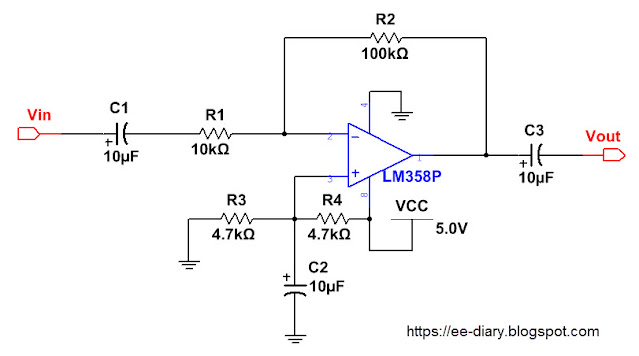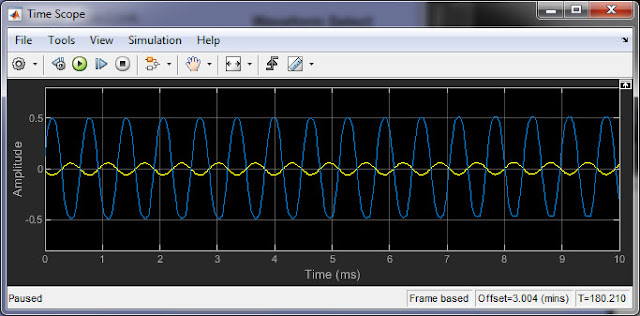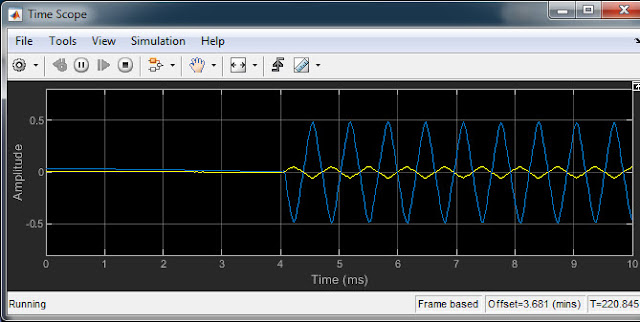# How to design a Practical LM358 Op-Amp Inverting Amplifier

Here we demonstrate how to design and test a practical LM358 op-amp inverting amplifier on a breadboard. The amplifier is tested with Matlab/Simulink based function generator and the input output signal waveforms are viewed on oscilloscope in real time. The input signal then interactively varied from a sine wave, square wave, triangle wave, sawtooth wave and pulse width modulated wave(PWM) of varying amplitude and frequency to analyze the response of the LM358 amplifier. The gain of the op-amp amplifier is set to 10 and a single supply voltage of 5V is used.

Following picture shows schematic diagram of the ac coupled Lm358 inverting amplifier.In the above circuit diagram, R2 is the inverting feedback resistor which together with the input resistor R1 provides the gain of the amplifier. The gain equation for inverting amplifier is,

$$A = - \frac{R_{2}}{R_{1}}$$

with $$R_{1}=10 K \Omega$$ and $$R_{2}=100 K \Omega$$, we get gain of A= -10 where -ve sign indicates that there is phase shift of 180 degree between the input and output signal(thats why called inverting amplifier).

The resistor R3 and R4 are used bias the voltage level in the mid range between supply Vcc=+5V and 0V. Using equal resistors R3=R4=4.7KOhm we get biased voltage at the non-inverting input as 2.5V and the signal amplitude at the output swings below and above 2.5V.

The capacitor C1 at the input and the output C3 are coupling capacitors. They are used to couple the signal at the input and to the output respectively. It allows ac signals to pass and blocks the dc signal. The cutoff frequency for the input coupling capacitor is given by,

$$f_{c1} = \frac{1}{2 \pi R_{1} C_{1}}$$

This cutoff frequency is chosen low as possible to let the low frequency components pass into the amplifier. With R1=10KOhm and C1=10uF we obtain cutoff frequency of $$f_{c1} = 1.59 Hz$$.

Similarly the output coupling capacitor C3 is used to block any dc and allow to pass ac signals. The cutoff frequency for this output coupling capacitor C3 is similarly calculated as before for input. The only thing required is the load resistance. In the above circuit diagram the load resistance or impedance is not shown. We will assume that the load resistance or impedance is 10KOhm then,

$$f_{c3} = \frac{1}{2 \pi R_{L} C_{3}}$$

As before if RL=10KOhm and C3=10uF then the cutoff frequency is  $$f_{c3} = 1.59 Hz$$.

The capacitor C2 is the bypass capacitor whose cutoff frequency is given by the following equation,

$$f_{c2} = \frac{1}{2 \pi (R_{3}|| R_{4}) C_{2}}$$

The parallel resistor equivalent of R3 and R4 is 2.35KOhm and substituting this value above with C2=10uF we get cutoff frequency as  $$f_{c2} = 6.77 Hz$$.

If the input signal is a sine wave with amplitude of 100mV and frequency of 1KHz and load resistor of 10KOhm is used at the output, then the input and output waveforms is shown below.

As you can see the output DC level 2.5V is removed by the output coupling capacitor C3. If we were to view input and output waveforms without the output coupling capacitor C3 we can see the output signal swings above and below 2.5V dc voltage.

The above LM358 Inverting Amplifier is assembled on a breadboard and is shown below.

### Testing & analysis LM358 amplifier with Matlab/Simulink PC Oscilloscope

To test and analyze the design of LM358 amplifier we will use Matlab/Simulink based PC oscilloscope. Before doing that we have to connect male to male jack to the PC speaker to the input of the amplifier to send various signal types and also connect with another male to male jack the output of the amplifier to the PC line in/microphone input to view the signal waveforms. The following picture shows how the amplifier is connected to the PC.

How this audio connection works is explained in more details in the tutorial How to build base biased BJT amplifier on breadboard and test with PC soundcard based oscilloscope and other similar tutorials which you can find at the end of this post. See also the tutorial How to use Matlab Simulink as Oscilloscope where simulink is used just to acquire signal and another PC software is used to generate waveforms.

The Matlab/Simulink based PC oscilloscope interface is shown below.

When we run the simulation, the matlab/simulink uses its audio blocks to send signals out from the speaker and we can collect signals from the PC line in/microphone port. We can select different types of waveforms to send out from the speaker. They are sine wave, triangle wave, sawtooth wave, square wave and PWM wave. With audio we can have two channels,left and right, both of which can be used to output and collect signals. We can turn on/off individual channels. We have vary the signal parameters such as the amplitude, frequency, phase and the duty cycle in case of pulse width modulated wave(PWM). However the frequency is limited to 22KHz because the PC audio hardware supports only audio signals to about 22KHz. Also the amplitude should be limited because the PC audio hardware only supports voltages on the order of mV. The prefboard picture above contains two potentiometer(left and right channel) and diodes with resistors that limits the voltage sensed by the line/in in order to protect that PC port.

See the following video demonstration of how it works.

The above video shows how we send different types of signals of different frequency and amplitude and how the output amplified signal are affected by the LM358 inverting amplifier. We can calculate the DC gain from the oscilloscope. The following picture shows input signals and the amplified output signals displayed on the time scope.

Sine waveTriangle WaveSawtooth wave

Square wave

PWM wave

We can analyze the frequency response using the Spectrum analyzer in real time. The following picture shows spectrum analyzer display.

With the use of Matlab Simulink PC oscillocope we can view the signal in real time in time and frequency domain. We can use cursor to measure the amplitude, noise etc. In the above spectrum analyzer display we can read the frequency contain in the signal in real time. Simulink spectrum analyzer has several good feature that helps us to automatically get the high frequency information and the magnitude in linear or dB.

In this way we can design an inverting amplifier using LM358 on a breadboard and test with Matlab/Simulink based PC oscilloscope. This is be useful to test any electronics circuit and no expensive high end oscilloscope is required. Also PC based oscilloscope is easy to use because we can use PC or laptop directly to analyze circuits instead of using oscilloscope which is heavy and requires one to move to laboratory.

We can also use other kinds of PC oscilloscope and test different kinds of circuits. Following links are some other methods to test circuits such as BJT amplifier using different kinds of PC oscilloscope and electronics design software.

- Testing of self biased BJT amplifier on breadboard with PC oscilloscope